Updating search results...

# 6 Results

View
Selected filters:
• Syllabus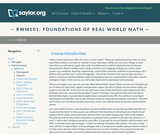Unrestricted Use
CC BY
Rating
0.0 stars

In this course, you will cover some of the most basic math applications, like decimals, percents, and even fractions. You will not only learn the theory behind these topics, but also how to apply these concepts to your life. You will learn some basic mathematical properties, such as the reflexive property, associative property, and others. The best part is that you most likely already know them, even if you did not know the proper mathematical terminology.

Material Type:
Activity/Lab
Full Course
Homework/Assignment
Syllabus
Provider:
The Saylor Foundation
08/28/2013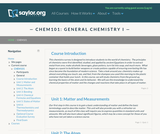Unrestricted Use
CC BY
Rating
0.0 stars

This survey chemistry course is designed to introduce students to the world of chemistry. In this course, we will study chemistry from the ground up, learning the basics of the atom and its behavior. We will apply this knowledge to understand the chemical properties of matter and the changes and reactions that take place in all types of matter. Upon successful completion of this course, students will be able to: Define the general term 'chemistry.' Distinguish between the physical and chemical properties of matter. Distinguish between mixtures and pure substances. Describe the arrangement of the periodic table. Perform mathematical operations involving significant figures. Convert measurements into scientific notation. Explain the law of conservation of mass, the law of definite composition, and the law of multiple proportions. Summarize the essential points of Dalton's atomic theory. Define the term 'atom.' Describe electron configurations. Draw Lewis structures for molecules. Name ionic and covalent compounds using the rules for nomenclature of inorganic compounds. Explain the relationship between enthalpy change and a reaction's tendency to occur. (Chemistry 101; See also: Biology 105. Mechanical Engineering 004)

Material Type:
Assessment
Full Course
Homework/Assignment
Lecture
Lecture Notes
Syllabus
Textbook
Provider:
The Saylor Foundation
11/16/2011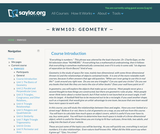Unrestricted Use
CC BY
Rating
0.0 stars

In this course, you will study the relationships between lines and angles. You will learn to calculate how much space an object covers, determine how much space is inside of a three-dimensional object, and other relationships between shapes, objects, and the mathematics that govern them.

Material Type:
Activity/Lab
Full Course
Homework/Assignment
Syllabus
Provider:
The Saylor Foundation
08/28/2013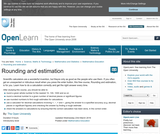Conditional Remix & Share Permitted
CC BY-NC-SA
Rating
0.0 stars

Scientific calculators are a wonderful invention, but they're only as good as the people who use them. If you often get an unexpected - or ridiculous - result when you press the"enter' button, this unit is for you. Learn how to do a calculation correctly and get the right answer every time.

Material Type:
Activity/Lab
Syllabus
Provider:
The Open University
Provider Set:
Open University OpenLearn
02/16/2011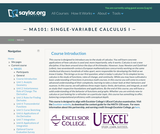Unrestricted Use
CC BY
Rating
0.0 stars

This course is designed to introduce the student to the study of Calculus through concrete applications. Upon successful completion of this course, students will be able to: Define and identify functions; Define and identify the domain, range, and graph of a function; Define and identify one-to-one, onto, and linear functions; Analyze and graph transformations of functions, such as shifts and dilations, and compositions of functions; Characterize, compute, and graph inverse functions; Graph and describe exponential and logarithmic functions; Define and calculate limits and one-sided limits; Identify vertical asymptotes; Define continuity and determine whether a function is continuous; State and apply the Intermediate Value Theorem; State the Squeeze Theorem and use it to calculate limits; Calculate limits at infinity and identify horizontal asymptotes; Calculate limits of rational and radical functions; State the epsilon-delta definition of a limit and use it in simple situations to show a limit exists; Draw a diagram to explain the tangent-line problem; State several different versions of the limit definition of the derivative, and use multiple notations for the derivative; Understand the derivative as a rate of change, and give some examples of its application, such as velocity; Calculate simple derivatives using the limit definition; Use the power, product, quotient, and chain rules to calculate derivatives; Use implicit differentiation to find derivatives; Find derivatives of inverse functions; Find derivatives of trigonometric, exponential, logarithmic, and inverse trigonometric functions; Solve problems involving rectilinear motion using derivatives; Solve problems involving related rates; Define local and absolute extrema; Use critical points to find local extrema; Use the first and second derivative tests to find intervals of increase and decrease and to find information about concavity and inflection points; Sketch functions using information from the first and second derivative tests; Use the first and second derivative tests to solve optimization (maximum/minimum value) problems; State and apply Rolle's Theorem and the Mean Value Theorem; Explain the meaning of linear approximations and differentials with a sketch; Use linear approximation to solve problems in applications; State and apply L'Hopital's Rule for indeterminate forms; Explain Newton's method using an illustration; Execute several steps of Newton's method and use it to approximate solutions to a root-finding problem; Define antiderivatives and the indefinite integral; State the properties of the indefinite integral; Relate the definite integral to the initial value problem and the area problem; Set up and calculate a Riemann sum; Estimate the area under a curve numerically using the Midpoint Rule; State the Fundamental Theorem of Calculus and use it to calculate definite integrals; State and apply basic properties of the definite integral; Use substitution to compute definite integrals. (Mathematics 101; See also: Biology 103, Chemistry 003, Computer Science 103, Economics 103, Mechanical Engineering 001)

Material Type:
Assessment
Full Course
Homework/Assignment
Syllabus
Textbook
Provider:
The Saylor Foundation
11/11/2011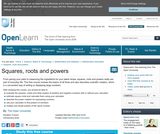Conditional Remix & Share Permitted
CC BY-NC-SA
Rating
0.0 stars

From paving your patio to measuring the ingredients for your latest recipe, squares, roots and powers really are part of everyday life. This unit reviews the basics of all three and also describes scientific notation, which is a convenient way of writing or displaying large numbers.

Material Type:
Activity/Lab